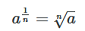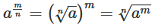# Rational Exponents Of A Real Number

## Number system of Class 9

### Principal of nth Root of a Positive Real Numbers :

If ‘a’ is a positive real number and ’n’ is a positive integer, then the principal nth root of a is the unique positive real number x such that xn = a.

The principal nth root of a positive real number a is denoted by a1/n.

Principal of nth Root of a Negative Real Numbers:

If ‘a’ is a negative real number and ‘n’ is an odd positive integer, then the principle nth root of a is define as -|a|1/n i.e. the principal nth root of -a is negative of the principal nth root of |a|.

Remark:

It ‘a’ is negative real number and ‘n’ is an even positive integer, then the principle nth root of a is not defined, because an even power of real number is always positive. Therefore (-9)1/2 is a meaningless quantity, if we confine ourselves to the set of real number, only.

### Rational Power (Exponents):

Radical expressions can also be written without using the radical symbol. We can use rational (fractional) exponents. The index must be a positive integer. If the index n is even, then a  cannot be negative.We can also have rational exponents with numerators other than 1. In these cases, the exponent must be a fraction in lowest terms. We raise the base to a power and take an nth th root. The numerator tells us the power and the denominator tells us the root.Rational exponents are another way to express principal nth th roots. The general form for converting between a radical expression with a radical symbol and one with a rational exponent is### LAWS OF RATIONAL EXPONENTS

The following laws hold the rational exponents

(i) am × an = am+n

(ii) a-m = 1/am

(ii) (am)n = am x n

(iv) am/an = am-n = 1/am-n

(v) (ab)n = a n. bn

(vi) a0 = 1

(vii) If am = an then m = n

(viii) If an = bn, a ≠ b, n = 0

Where a,b are positive real number and m,n are relational numbers.